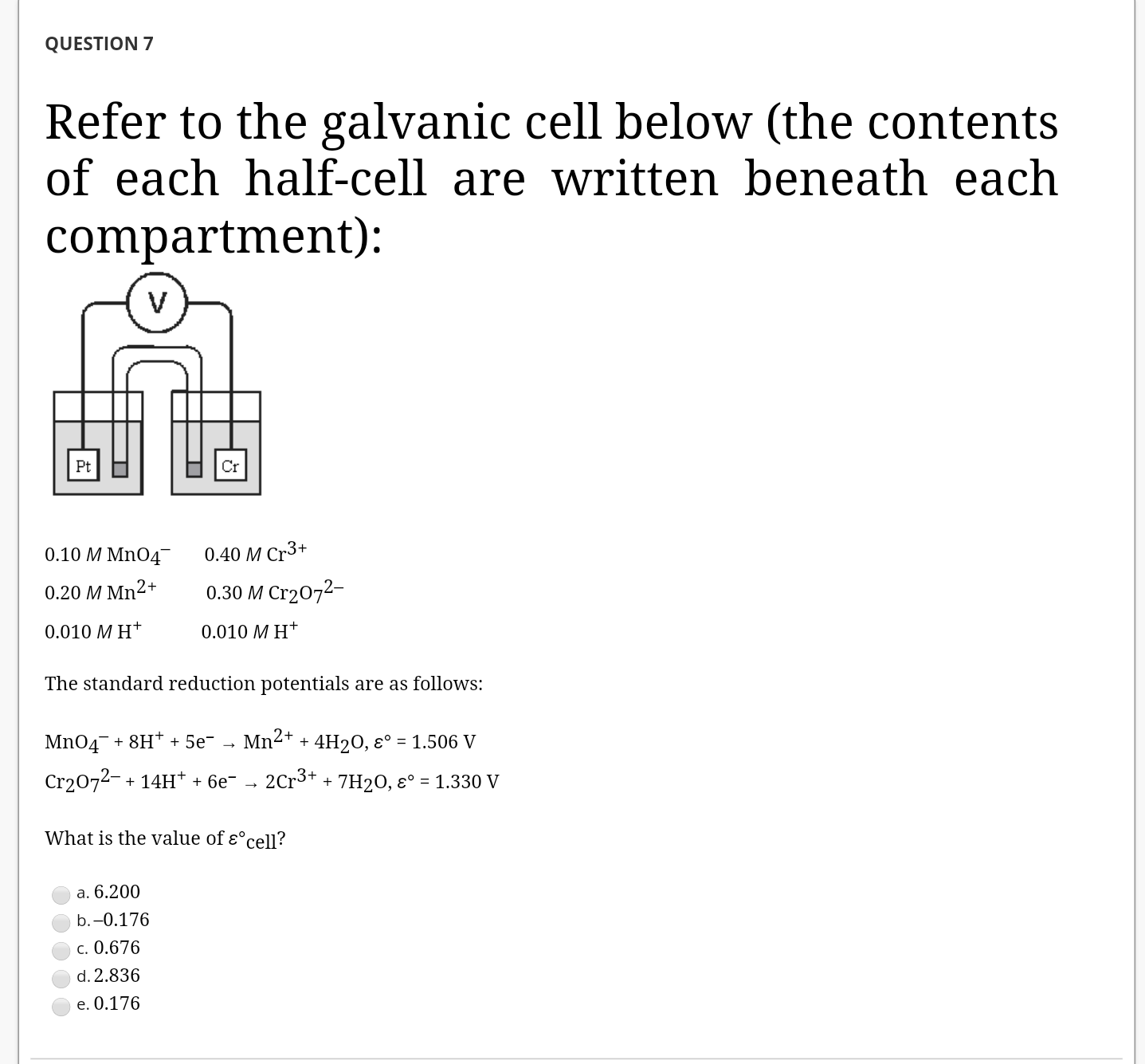# Refer to the galvanic cell below (the contents of each half-cell are written beneath each compartment): The standard reduction potentials are as follows: MnO4- + 8 H+ + 5 e- → Mn2+ + 4 H2O E = 1.506 V Cr2O72- + 14 H+ + 6 e- → 2 Cr3+ + 7 H2O E = 1.330 V What is the value of ecell ?